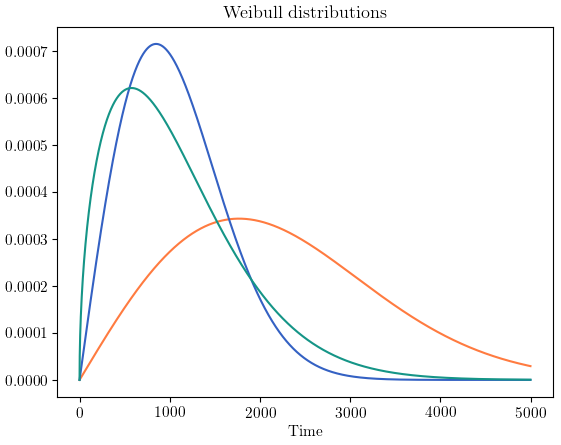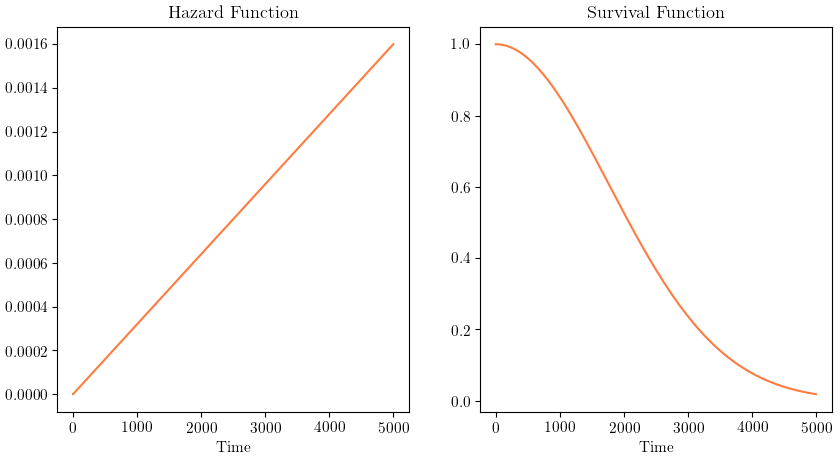# Survival Analysis, Part 1: The Weibull model

Learn more about the fundamentals of survival models, how observed data can be incorporated to improve predictive maintenance, and how the data can be used to train survival models.This introductory series of posts is meant to serve as a high-level overview of survival modeling in the context of machine failure prediction. We’ll be covering the basics of survival models, how observed historical data can be incorporated to improve predictive performance, and how this observed data can be used to train survival models. Specifically, this post provides a high-level introduction to:

• Survival analysis
• The Weibull model
• Fitting Weibull models given observed data

Questions, suggestions and feedback are welcome and can be directed to the author.

# Survival Analysis

Survival analysis is a branch of statistics designed for analyzing the expected duration until an event of interest occurs. In general, our “event of interest” is the failure of a machine. We denote the time at which this machine fails by T, where T>0 (Time 0 denotes the time at which the machine was installed). The Weibull distribution is particularly popular in survival analysis, as it can accurately model the time-to-failure of real-world events and is sufficiently flexible despite having only two parameters. We use the Weibull distribution to model the distribution of failure times for a fleet of machines.Example Weibull distributions. These can be used to model machine failure times.

One crucially important statistic that can be derived from the failure time distribution is the hazard function, h(t). The hazard function represents the probability of failure in the next time period t+1, given the asset has survived up until time t. The mathematical formulation is then:

h(t) = Pr(T = t+1|T>t)

A great variety of statistics can be derived from the hazard function for a particular asset, including:

• Expectation of remaining useful life (RUL)
• RUL variance and higher-order statistical moments such as skewness and kurtosis
• Probability of failure at a specific time t
• Probability of failure within a certain time interval
• Probability of survival up until a given time

The last of these, known as the survival function S(t), merits special attention as it is one of the most useful and intuitive statistics associated with survival analysis. The survival function is simply the probability that a machine will fail after a certain time t, or equivalently that it will still be in service at time t.

S(t) = Pr(T>t)Hazard and survival functions for a hypothetical machine using the Weibull model.

As time goes on, it becomes more and more likely that the machine will fail in the next period given that it has lived until the current period; therefore the hazard function in the example above steadily increases. And it becomes less likely that the machine will still be alive after each successive period, which is why the survival function for the same hypothetical machine decreases as time goes on.

# Fitting a Weibull model to data

This all seems somewhat abstract. What exactly is the connection between the Weibull model described above and machine learning? Concretely, we would like to use observed data in order to predict the remaining life for each of a group of machines. To understand how this can be done, it’s easiest to build up a model piece by piece. The minimum amount of data we require to build a predictive model are the observed lifetimes of machines that have failed.

Imagine Company X maintains a fleet of 10,000 machines that are known to be failure-prone. They’re pretty thorough about record-keeping and maintain records of each machine’s installation and failure time, making it easy to determine how long each machine was in service before it failed. 100 of their machines have failed unexpectedly so far, and they decide they’ve had enough. By making a simple histogram, they’re able to see that most of their machines fail between one and ten years after being put into service:

If only this observed distribution of machine lifetimes could be approximated by some sort of model that would allow us to make useful predictions…

As the astute reader may have guessed, we can fit the Weibull model to this data. There are exactly two parameters, known as the shape and the scale parameters. We can find the shape and scale parameters that best fit the data (according to a specific definition of “best”) using a method known as maximum likelihood. Maximum likelihood basically says: given the observed data, which possible version of the Weibull distribution is it most likely that the data came from? After finding the optimal shape and scale parameters, we plot the histogram again with the fit Weibull model superimposed:

As we had hoped, the optimized Weibull model approximates the distribution of failure times reasonably well. It doesn’t fit the data perfectly, but this isn’t that surprising — it only has two “knobs” (parameters) that can be adjusted in order to determine its exact configuration. But from this seemingly simple distribution, we can now compute:

• Hazard function
• Survival function